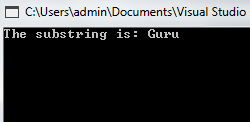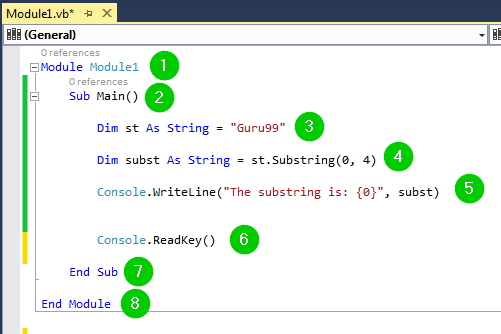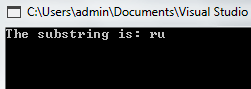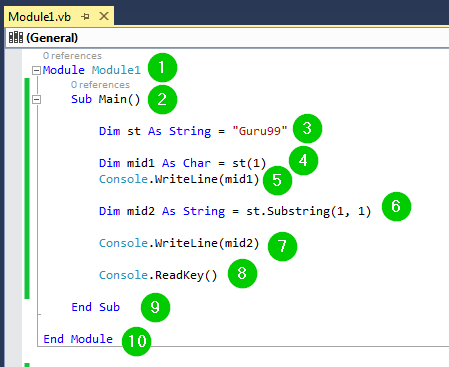# VB.NET Substring Method with EXAMPLE

## What is a SubString?

The substring function is used to obtain a part of a specified string. This method is defined in the String class of Microsoft VB.NET. You have to specify the start index from which the String will be extracted. The String will be extracted from that index up to the length that you specify.

In this tutorial, you will learn

## Syntax of Substring

The function accepts two arguments as shown in the following syntax:

```Public Function Substring(ByVal start_Index As Integer, ByVal sub_length As Integer) As String
```

Here,

• The ByVal keyword denotes pass-by-value, which is a mechanism of passing arguments to functions.
• The start_Index is the index from which the substring will be obtained
• sub_length denotes the length up to which the String will be copied from the start_Index. This length is measured in terms of the number of characters. The function will return the extracted substring.

## Examples

Step 1) Create a new console application.

Step 2) Add the following code to it:

```Module Module1
Sub Main()

Dim st As String = "Guru99"

Dim subst As String = st.Substring(0, 4)

Console.WriteLine("The substring is: {0}", subst)

End Sub

End Module
```

Step 3) Click the Start button from the toolbar to execute the code. You should get the following result:We have used the following code:Explanation of Code:

1. Creating a module named Module1.
2. Starting the main sub-procedure.
3. Defining a string variable named st and assigning the value Guru99 to it.
4. Defining a string variable named ‘subst’ as a substring of the String ‘st’ from index 0f and a length of 4 characters.
5. Printing some text and the above substring on the console.
6. Pausing the console window for a while waiting for the user to take action to close it.
7. End of the main sub-procedure.
8. End of the module.

## One Argument

What if we pass only one argument to the function? The function will copy all the data in the String that begins from that index. What happens is that the Substring function internally copies all the string data at that index as well as that which follows that index. For example:

```Module Module1
Sub Main()

Dim st As String = "Guru99"

Dim subst As String = st.Substring(4)

Console.WriteLine("The substring is: {0}", subst)

End Sub

End Module
```

Click the Start button to run the code. It should return the following:The substring function returned 99. We passed the parameter 4 to the function, meaning that it will begin to extract the substring from the character at index 4 to the end of the String. 9 is the character at index 4 of the string Guru99, hence the extraction started there.

## Middle Characters

It is also possible for us to get the middle characters of the String in question. In this case, we only have to provide the starting index and the length of the String that we need. In the following example, we are getting a substring of the specified String from index 2 and the String will have a length of 2 characters:

```Module Module1
Sub Main()

Dim st As String = "Guru99"

Dim subst As String = st.Substring(2, 2)

Console.WriteLine("The substring is: {0}", subst)

End Sub

End Module
```

Click the Start button from the toolbar to run the code. You will get the following result:In the above example, the substring function returned ru. We passed the parameters (2, 2) to the function. The first 2 instructs the function to begin the extraction of the substring from index 2 while the second 2 instructs the function to return a substring with a length of 2 characters only. This means that the extraction of the substring should begin from the element located at index 2 of the string Guru99, which is r. Since the returned substring should only have a length of 2 characters, the extraction will not go past the ‘u’, hence it returned ‘ru.’

## One Char

We can use the Substring function to get a single character from a string. In such a case, it is a necessity for you to make an allocation but the character can be accessed directly. This is a bit faster. The following example demonstrates two ways through which we can achieve this:

```Module Module1
Sub Main()

Dim st As String = "Guru99"

Dim mid1 As Char = st(1)
Console.WriteLine(mid1)

Dim mid2 As String = st.Substring(1, 1)

Console.WriteLine(mid2)

End Sub

End Module
```

Click the Start button to run the code. You will get the following result:We have used the following code:Explanation of Code:

1. Creating a module named Module1.
2. Starting the main sub-procedure.
3. Defining a string variable named st and assigning the value Guru99 to it.
4. Defining a string variable named mid1 and getting the character at index 1 of String st. This character will be assigned to the variable mid1.
5. Printing the above character on the console.
6. Defining a string variable named mid2 and getting the character at index 1 with a length of 1 from String st. The length of 1 means that it will return the same character at the starting index. The counting begins from the starting index that you specify. This character will be assigned to the variable mid2.
7. Printing the above character on the console.
8. Pausing the console window for a while waiting for the user to take action to close it.
9. End of the main sub-procedure.
10. End of the module.

## Summary

• The Substring function is defined in the String class of Visual Basic.NET.
• It accepts two arguments, which is the starting point of the substring and the length of the substring.
• We can play around with these arguments to get various sets of substrings from the main String.

#### Top Courses